## The index of refraction of a clear plastic is listed as 1.89 in the book, but you measured the angle of incidence 63.5° and the angle of ref

Question

The index of refraction of a clear plastic is listed as 1.89 in the book, but you measured the angle of incidence 63.5° and the angle of refraction 32°. What index of refraction did you calculate and what is the percentage error?

in progress 0
4 weeks 2021-08-19T13:01:11+00:00 1 Answers 1 views 0

## percentage error = 10.58%

Explanation:

According to Snell’s law, the ratio of the sine of angle of incidence to the sine of angle of refraction is a constant for a given pair of media. The constant is known as the refractive index.

Mathematically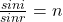i = angle of incidence measured = 63.5°

r = angle of refraction measured = 32°

n = refractive index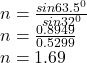The index fraction calculated is approx. 1.69.

If the index of refraction of a clear plastic as listed in the book is 1.89 and the calculated is 1.69, the percentage error will be calculated as thus;

%error =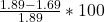%error =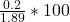%error  =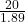%error  = 10.58%